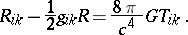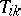# Einstein equations

(diff) ← Older revision | Latest revision (diff) | Newer revision → (diff)
Jump to: navigation, search

of the gravitational field

Fundamental equations in the general theory of relativity. They connect the metric tensor of the space-time continuum, which describes the gravitational field, and the physical characteristics of different forms of matter, described by means of the energy-momentum tensor:Hereis the Ricci tensor, which can be expressed in terms of the metric tensor,,is the energy-momentum tensor,is the speed of light in vacuum, andis the gravitational constant.